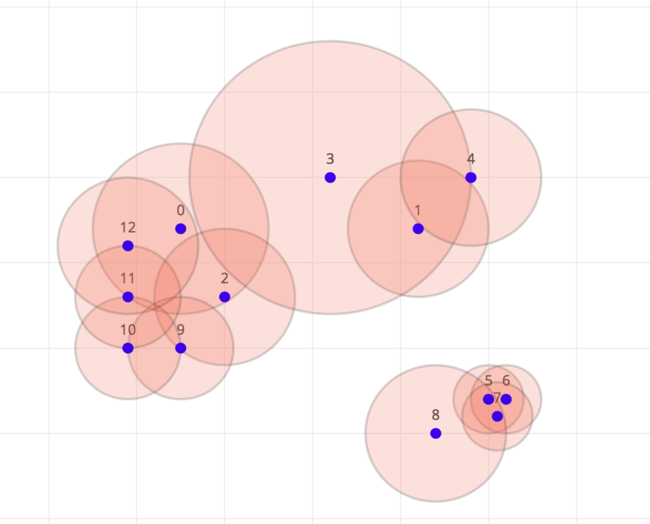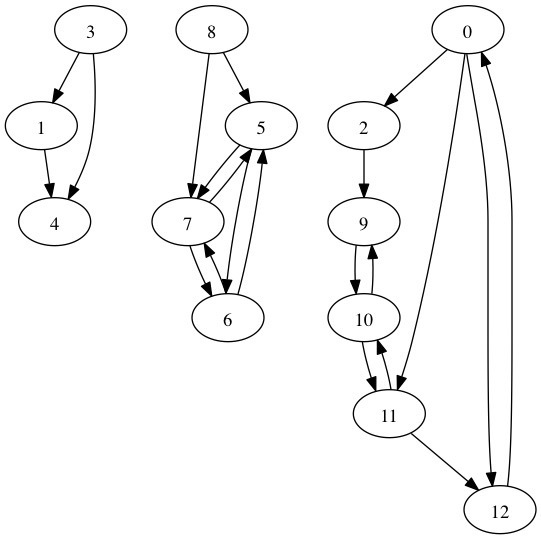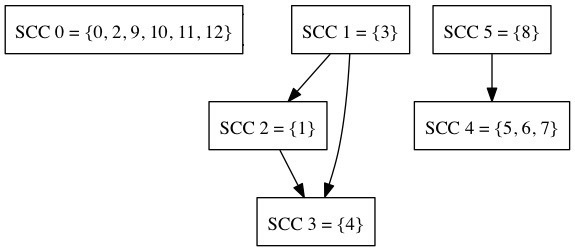# Mines Chain Reaction

This post is based on a question asked during the Challenge 24 programming competition. Given the locations of a number of land mines as `X` and `Y` coordinates and their blast radius `R`. What is the minimum number of mines that need to be detonated such that all mines are detonated. When a mine is detonated it detonates all mines within its blast radius and the process repeats.Here’s a simple example with `13` mines. In this case the optimal solution is to detonate mines `0, 3` and `8` which will detonate all others. It’s not the only solution.The relationship between mines is not commutative. Just because mines `A` can reach mine `B` doesn’t mean that mine `B` reaches mine `A`. Therefore we can represent the mines as a directed graph where vertices are mines and there is an (unweighted) edge from mine `A` to mine `B` if mine `A` can directly detonate mine `B`.In order to solve this problem we first need to compute the strongly connected components in this graph . These are the subsets of mines which if any one is detonated then all mines in the subset will be detonated. In the example image above mines `5, 6`, and `7` comprise a SCC as do mines `0, 2, 9, 10, 11` and `12`. For simplicity we’ll say that mines on their own are also in SCCs of size `1`. In order to compute the SCCs we can use Tarjan’s algorithm which can be implemented recursively or with a stack.

``````def tarjan(graph):

index_counter = 
stack = []
index = {}
result = []

def strongconnect(node):
# depth index for this node
index[node] = index_counter
index_counter += 1
stack.append(node)

# Consider successors of `node`
try:
successors = graph[node]
except:
successors = []
for successor in successors:
# Successor has not yet been visited
strongconnect(successor)
elif successor in stack:
# the successor is in the stack

# pop the stack and generate an SCC
connected_component = []

while True:
successor = stack.pop()
connected_component.append(successor)
if successor == node: break
component = tuple(connected_component)
# storing the result
result.append(component)

for node in graph:
strongconnect(node)

return result
``````

This computes the SSCs for the initial graph. Now we can collapse all vertices in a SCC into a super vertex. Remember detonating any mine in the super vertex will detonate all the other in that super vertex. Then we can create another graph of the super vertices and connect super vertices with a directed edge if any mine in that super vertex can detonate any mine in another super vertex. We now get another directed graph although this one won’t have cycles. Remember if we denote a mine in a connected component it will detonate all mines in that component and all mines in all components reachable from that support node. Here’s an illustration to or the process so far:We can now work out which mines need to be detonated. In order to do this we can look for all super vertices in this graph that have a zero in-degree. This means that they aren’t reachable by any sequence of mine detonations and thus need to be detonated themselves. One solution is to detonate mines: `0, 3` and `8`. There are actually multiple solutions. We can see this for example by considering the case where all the mines are within blast radius of all others and thus form one strongly connected components. In this case we could choose any mine to start the chain reaction.

In the competition the test cases got really large. The smallest had `500` vertices and the largest had `800,000` vertices. Tarjan’s algorithm is really fast and runs in `O(N)`. Similarly the degree counting can also be done in `O(N)`. The slowest part it actually creating the initial graph which when done naively takes `O(N^2)`. In order to process the larger test cases we need to use a range query structure like a KD-Tree to query all mines within `R` of a specific mine in logarithmic time. Reducing the processing to `O(N log N)`. A simpler approach than implementing a KD-Tree is to sort all the mines by their `X` coordinate and only consider partner mines that are within `X*X` < `R*R` of original. With randomly spaced data this gets you close to `O(N log N)` without too much more coding. The problem set is available here.

This type of analysis is useful in considering the distribution of information through a network. If the initial graph represented people and edges represented the people with whom they shared information. Then the source nodes are the minimum set of people that need to be given information such that it is transferred through the whole network.